# Mathematical description of law of motion

## NEWTON’S FIRST LAW OF MOTION:

“Everybody continues to be in a state of rest or uniform motion in a straight line, except external force acts on the body under observation.”

### Conclusion:

The above law gives us an idea that it is a property of a body to maintain its state of rest or motion. This property is called as inertia. Note that inertia cannot be measured as it is merely a property. However inertia of a body increases with the increase of the mass of the body.

### NEWTON’S SECOND LAW OF MOTION:

This law states “The rate of change of momentum of a body is directly proportional to the force applied on the body and change in direction of the momentum takes place in the direction of the force”.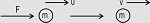Let a force F acts on a body of mass m moving with a velocity u. Let after some time t the velocity changes to v.

Here, the quantity mu is initial momentum and mv is the final momentum.

Therefore, change in momentum = mv – mu

Hence, rate of change in momentum = (mv – mu) / t

According to the second law,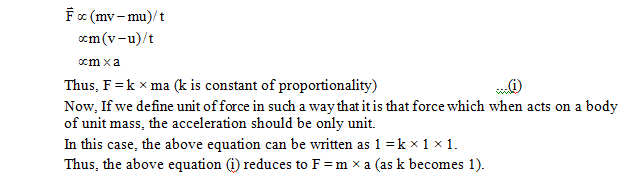### NEWTON’S THIRD LAW OF MOTION:### Principle of conservation of momentum:

Let a body of mass m1 moving with a velocity u1 and another body of mass m2 moving with a velocity u2. Let u1 > u2.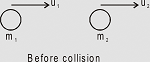Obviously m1 will collide with m2. Let after collision velocity of m2 becomes v1 and velocity of m2 becomes v2. During collision m1 will exert force on m2 and vice-versa.

The force exerted by m1 on m2,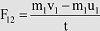And the reaction force of m2 on m1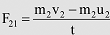Now, according to third law of motion F12 = – F21

or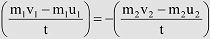or    m1 u1 + m2 u2 = m1 v1 + m2 v2

Total momentum before collision = the total momentum after the collision.

Thus, we can conclude that during coillision of two bodies the total momentum remain constant before and after the collision provided no external force acts on them. This is known as principle of conservation of momentum.

### FREE BODY DIAGRAM:

No system, natural or manmade consists on a single body along or is complete in itself. A single body or a part of the system can, however, be isolated from the rest by appropriately accounting for its effect on the remaining system.

A free body diagram (FBD) consists of a diagrammatic representation of a single body or a subsystem of bodies isolated from its surroundings showing all the forces acting on it.

Consider, for example, a book lying on a horizontal surface.

The following examples show the free body diagrams of different bodiesTo draw the free body diagram of anybody keep in mind the following points.

• a.    We have to represent weight for everybody. (If we assume pulleys are massless then we will not represent weight)
• b.    Observer whether the body is in contact with the other body (surface). If it is in contact with any surface then we have to represent normal reaction force and frictional force (if any).
• c.    If there is any string attached with the body then we have to represent tension force.

Solved Examples

Ex.1   Name the unbalanced force that stops a ball rolling on the ground.

Sol.   Force of friction between the ball and the ground.

Ex.2   A rubber ball is pressed. Which force: balanced or unbalanced, is applied?

Sol.   The force applied is balanced force.>

Ex.3   Give two examples each of situations in which you push or pull to change the state of motion of objects.

Sol.

• (i)    Moving a book.
• (ii)    Pushing the moving ball by a volleyball player to his team-mate.

Ex.4   When we talk of force acting on a body, do we mean unbalanced force or balanced force?

Sol.   Force acting on a body usually means an unbalanced force.

Ex.5    Name the various effects of force.

Sol.    Force may

• (i)      move a stationary body.
• (ii)      stop a moving body.
• (iii)      change the direction of motion of the body.
• (iv)      change the shape and size of the body.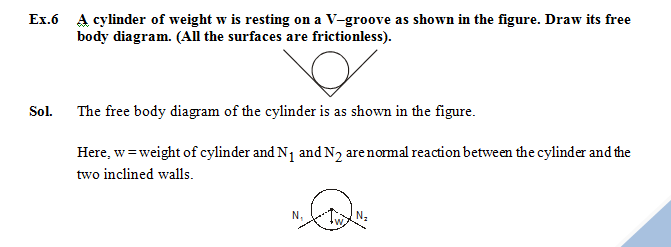Here, w = weight of cylinder and N1 and N2 are normal reaction between the cylinder and the two inclined walls.

Ex.7   Three blocks A, B and C are placed one over the other as shown in the figure. Draw free body diagrams of all the three blocks. (All the surfaces are frictionless).